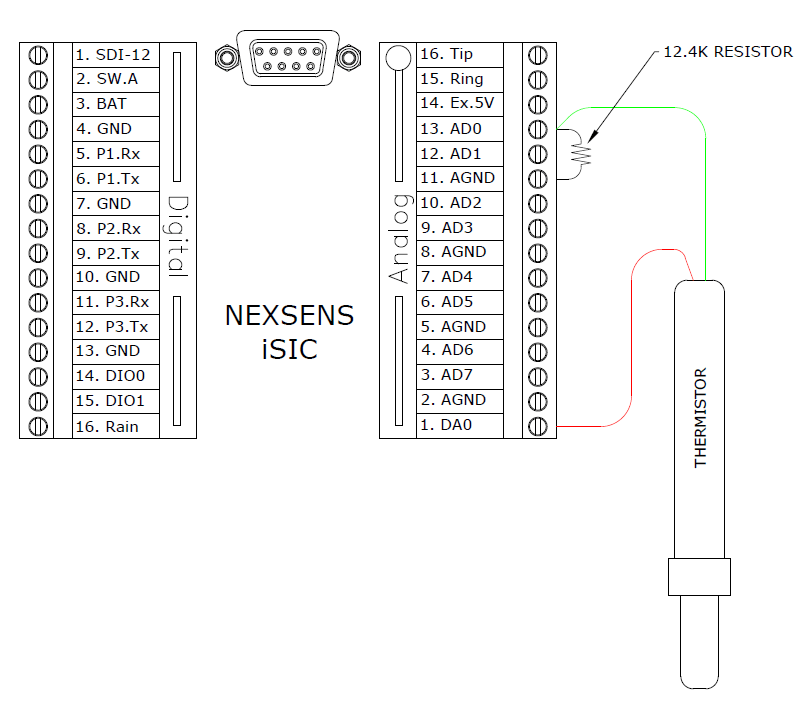A thermistor can be connected to the iSIC through the analog terminal strip. Follow the connection diagram below to properly connect a thermistor. The iSIC utilizes the Steinhart-Hart equation for calculating temperature.Figure 1: Thermistor

A single resistor across AGND and the analog input pin should be used to complete the equation. The resistor size should be around the same size as the thermistor resistance at 25 C. For example, a 10K Thermistor could use a 12.4K resistor. A 100K Thermistor could use a 100K resistor. One thermistor can be connected to each analog input pin.

REV: 13G02